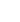15min:
COMPUTING ROVIBRATIONAL LEVELS OF POLYATOMIC MOLECULES WITH CURVILINEAR INTERNAL VIBRATIONAL COORDINATES AND AN ECKART FRAME.

XIAO-GANG WANG AND TUCKER CARRINGTON JR., Chemistry Department, Queen's University, Kingston, Canada.

\noindent We present a new procedure for computing a rovibrational spectrum of a polyatomic molecule. The Schrödinger equation is solved, numerically exactly, by using a nested contracted basis. Rovibrational wavefunctions are computed in a | v \rangle \vert JKM \rangle basis, where | v \rangle is a vibrational wavefunction and \vert JKM \rangle is a symmetric top wavefunction. In turn, the | v \rangle are obtained by solving a vibrational Schrödinger equation with basis functions that are products of contracted bend and stretch functions. At all stages of the calculation we exploit parity symmetry. The calculations are done in internal coordinates that facilitate the treatment of large amplitude motion. An Eckart molecule-fixed frame is used by numerically computing coefficients of the kinetic energy operator.

The method is significantly more efficient than the previous method which uses a molecule-fixed frame attached to two vectors and vibrational basis functions that depend on K.

% The efficacy of the method is demonstrated by calculating a large number of converged J=10 methane rovibrational levels in the Tetradecad polyad (vibrational energies in the range 5100 - 6100 cm-1). No previous calculation of rovibrational levels of methane includes as many levels as we report in this paper.